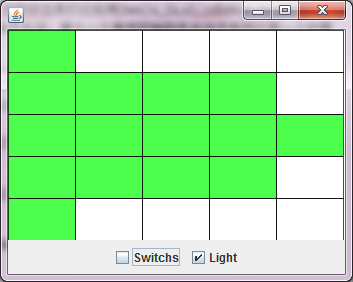Lights Out(关灯游戏) 是一款经典的老游戏，维基百科上说Lights Out 是在1995年由 Tiger Toys 第一次发行。接触它却在去年的时候Gnome3的小游戏里。Lights Out 的规则很简单，游戏由一个 5 * 5 格的棋盘构成，每格子都是一颗灯，灯肯定是有两种状态，亮或者暗。游戏一开始棋盘上会亮起一些灯，你的目标就是把所有亮起灯都关掉，亮起的灯点一下就会关掉，但游戏当然不会这么简单，点一下灯的时候还会有一些附加影响，准确的说就是点一下灯的时候会切换它和四周也就是是上下左右的灯的状态。找到了一个在线的Lights Out，http://www.addictinggames.com/puzzle-games/lightsout.jsp。一般能过第五关就差不多了吧。$\begin{vmatrix}1 & 0 & 0 & 0 & 0 \\1 & 1 & 1 & 1 & 0 \\1 & 1 & 1 & 1 & 1 \\1 & 1 & 1 & 1 & 0 \\1 & 0 & 0 & 0 & 0\\\end{vmatrix}$

$\vec s_{1,1}=(1,1,0,0,0,1,0,\cdots,0)$

$\vec s_{1,2}=(1,1,1,0,0,0,1,\cdots,0)$

$$\vec s_{5,5}=(0,\cdots,0,1,0,0,0,1,1)$$ 。

$$\vec x=(x_{1,1},x_{1,2},\cdots,x_{1,5},x_{2,1},\cdots,x_{5,5})^T$$ 。由于游戏的开关状态时未知的，是我们玩这个游戏的目的，所以这里用x表示。$$\vec x$$即是初始局面打开的开关，也是灯全暗变换到棋盘初始状态所需要点开的开关，所以我们也把$$\vec x$$称为一个策略。$$\vec b$$表示初始状态，$$\underline{0}$$灯光全暗的状态。

$\vec b =\underline{0}+x_{1,1}\vec s_{1,1}+\cdots+x_{1,5}\vec s_{1,5}+x_{2,1}\vec s_{2,1}+\cdots+x_{5,5}\vec s_{5,5}$

$A = \begin{vmatrix}B & I & O & O & O \\I & B & I & O & O \\O & I & B & I & O \\O & O & I & B & I \\O & O & O & I & B \\\end{vmatrix}$

I是一个 5 * 5 的单位矩阵，O是一个 5 * 5 其元全部为零的矩阵。而B是这样一个矩阵：

$B = \begin{vmatrix}1 & 1 & 0 & 0 & 0 \\1 & 1 & 1 & 0 & 0 \\0 & 1 & 1 & 1 & 0 \\0 & 0 & 1 & 1 & 1 \\0 & 0 & 0 & 1 & 1 \\\end{vmatrix}$

$\vec x_{1}=(0,1,1,1,0,1,0,1,0,1,1,1,0,1,1,1,0,1,0,1,0,1,1,1,0)^T$ $\vec x_{2}=(1,0,1,0,1,1,0,1,0,1,0,0,0,0,0,1,0,1,0,1,1,0,1,0,1)^T$

keyboard_arrow_up